# KSEEB Solutions for Class 9 Maths Chapter 7 Quadrilaterals Ex 7.2

KSEEB Solutions for Class 9 Maths Chapter 7 Quadrilaterals Ex 7.2 are part of KSEEB Solutions for Class 9 Maths. Here we have given Karnataka Board Class 9 Maths Chapter 7 Quadrilaterals Exercise 7.2.

## Karnataka Board Class 9 Maths Chapter 7 Quadrilaterals Ex 7.2

Question 1.
ABCD is a quadrilateral in which P, Q, R and S are mid-points of the sides AB, BC, CD and DA. AC is a diagonal. Show that :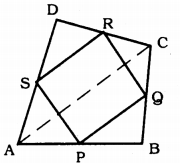(i) SR || AC and SR = $$\frac{1}{2}$$ AC
(ii) PQ = SR
(iii) PQRS is a parallelogram.
Solution:
Data: ABCD is a quadrilateral in which P, Q, R, and S are mid-points of the sides AB, BC, CD and DA. AC is a diagonal.
To Prove: (i) SR || AC and SR = $$\frac{1}{2}$$ AC
(ii) PQ = SR
(iii) PQRS is a parallelogram.
Proof: (i) In ∆ADC, S, and R are midpoints of AD and DC sides.
As per mid-point theorem,
SR || AC
and SR = $$\frac{1}{2}$$ AC.

(ii) In ∆ABC, P and Q are mid-points of AB and BC.
As per mid-point theorem,
PQ || AC
and PQ = $$\frac{1}{2}$$ AC
But, SR = $$\frac{1}{2}$$ AC (Proved)
∴ PQ = SR

(iii) PQ = SR (Proved)
SR || AC and PQ || AC
∴ SR || PQ
Opposite sides of a quadrilateral PQRS are equal and parallel, hence PQRS is a parallelogram.

Question 2.
ABCD is a rhombus and P, Q, R, and S are the mid-points of the sides AB, BC, CD, and DA respectively. Show that the quadrilateral PQRS is a rectangle.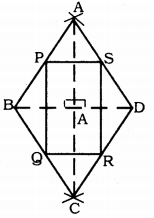Solution:
Data: ABCD is a rhombus and P, Q, R, and S are the mid-points of the sides AB, BC, CD, and DA respectively.
To Prove: PQRS is a rectangle.
Construction: Diagonals AC and BD are drawn.
Proof: To prove PQRS is a rectangle, one of its angles should be the right angle.
In ∆ADC, S and R are the midpoints of AD and DC.
∴ SR || AC
SR = $$\frac{1}{2}$$ AC (mid-point formula)
In ∆ABC, P and Q are the midpoints AB and BC.
∴ PQ || AC
PQ = $$\frac{1}{2}$$ AC.
∴ SR || PQ and SR = PQ
∴ PQRS is a parallelogram.
But diagonals of a rhombus bisect at right angles. 90° angle is formed at ’O’.
∴ ∠P = 90°
∴ PQRS is a parallelogram, each of its angles is a right angle.
This is the property of the rectangle.
∴ PQRS is a rectangle.

Question 3.
ABCD is a rectangle and P, Q, R, and S are mid-points of the sides AB, BC, CD, and DA respectively. Show that the quadrilateral PQRS is a rhombus.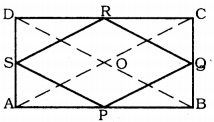Solution:
Data: ABCD is a rectangle and P, Q, R, and S are mid-points of the sides AB, BC, CD, and DA respectively.
To Prove: PQRS is a rhombus.
Construction: Diagonals AC and BD are drawn.
Proof: In ∆ABC, P and Q are the mid-points of AD and BC.
∴ PQ || AC (Mid-point theorem)
PQ = $$\frac{1}{2}$$ AC ………….. (i)
Similarly, in ∆ADC, S and R are the mid-points of AD and CD.
∴ SR || AC
SR = $$\frac{1}{2}$$ AC …………… (ii)
Similarly, in ∆ABD,
SP || BD
SP = $$\frac{1}{2}$$ BD ……………….. (iii)
Similarly, in ∆BCD,
QR || BD
QR = $$\frac{1}{2}$$ BD ……………… (iv)
From (i), (ii), (iii) and (iv),
PQ = QR = SR = PS and Opposite sides are parallel.
∴ PQRS is a rhombus.

Question 4.
ABCD is a trapezium in which AB || DC, BD is a diagonal and E is the mid-point of AD. A line is drawn through E parallel to AB intersecting BC at F. Show that F is the mid-point of BC.Solution:
Data: ABCD is a trapezium in which AB || DC, BD is a diagonal, and E is the mid-point of AD.
A line is drawn through E parallel to AB intersecting BC at F.
To Prove: F is the mid-point of BC.
Proof: Straight line EF cuts at G.
In ∆ABD, E is the mid-point of AD (Data)
EF || AB
EG || AB
∴ G is the mid-point of BD.
∵ Converse of mid-point formula.
DC || AB and EF || AB
⇒ DC || EF
In ∆BDC,
GF || DC
G is the mid-point of BD (proved)
∴ F is the mid-point of BC.

Question 5.
In a parallelogram ABCD, E and F are the mid-points of sides AB and CD respectively. Show that the line segments AF and EC trisect the diagonal BD.Solution:
Data: In a parallelogram ABCD, E and F are the mid-points of sides AB and CD respectively.
To Prove: Line segments AF and EC trisect the diagonal BD.
Proof: ABCD is a parallelogram.
AB || DC and AB = DC
$$\frac{1}{2}$$ AB = $$\frac{1}{2}$$ DC
AE = CF
and AE || CF (∵ AB || CD)
∴ AE || CF and AE = CF.
∴ AECF is a parallelogram.
∴ AF || EC
In ∆DQC, PF || QC (∵ AF || EC)
∴ P is the mid-point of DQ.
∴ DP = PQ ………….. (i)
In ∆APB, EQ || AP.
But E is the mid-point of AB (Data)
∴ Q is the mid-point of PB.
∴ PQ = QB …………… (ii)
From (i) and (ii),
DP = PQ = QB
∴ AF and EC line segments trisect the diagonal BD.

Question 6.
Show that the line segments joining the mid-points of the opposite sides of a quadrilateral bisect each other.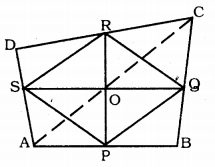Solution:
Data : P, Q, R and S are mid-points of AB, BC, CD and DA respectively in quadrilateral ABCD.
To Prove: PR and SQ line segments bisect mutually.
Construction: Join the diagonal AC.
S and R are the mid-points of AD and DC.
∴ SR || AC
SR = $$\frac{1}{2}$$ AC ………… (i) (Mid-point Theorem)
Similarly, in ∆ABC,
PQ || AC
PQ = $$\frac{1}{2}$$ AC …………. (ii)
From (i) and (ii),
SR = PQ and SR || PQ
∴ PQRS is a parallelogram.
PR and SQ are the diagonals of parallelogram PQRS.
∴PR and SQ meet at O’.

Question 7.
ABC is a triangle right angled at C. A line through the mid-point M of hypotenuse AB and parallel to BC intersects AC at D. Show that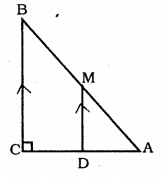(i) D is the mid-point of AC.
(ii) MD ⊥ AC
(iii) CM = MA = $$\frac{1}{2}$$AB.
Solution:
Data: ABC is a triangle right angled at C. A line through the mid-point M of hypotenuse AB and parallel to BC intersects AC at D.
To Prove: (i) D is the mid-point of AC.
(ii) MD ⊥ AC
(iii) CM = MA = $$\frac{1}{2}$$ AB.
Proof: (i) In ∆ABC,
M is the mid point of AB and
MB || BC Mid-point of BC (Data)
∴ D is the mid-point of AC (Mid-point formula)

(ii) MD || BC
∠BCD + ∠MDC = 180° (Sum of interior angles)
90 + ∠MDC = 180°
∠MDC = 180 – 90
∠MDC = 90°
∴ MD ⊥ AC

(iii) In this fig. CM is joined.
M is the mid-point of AB.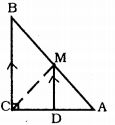∴ MA = $$\frac{1}{2}$$ AB ……….. (i)
Now, in ∆AMD and ∆CMD.
CM = MA = $$\frac{1}{2}$$ AB.